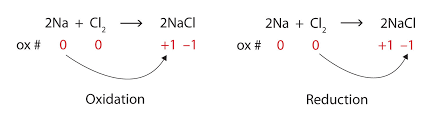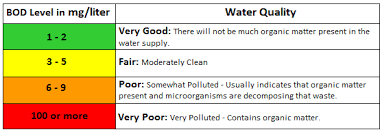1.  Oxidation and Reduction

Oxidation and reduction can be considered in terms of:

• oxygen gain or hydrogen loss,
• electron transfer OR
• change in oxidation number

Consider the following reaction:

Zn(s)  +  Cu2+(aq)  –>  Zn2+(aq)  +  Cu(s)

Take a look below for a model of what is happening on an atomic level.

✍️  Looking at the change in charges, which species is gaining electrons and which species is losing electrons?

✍️  Define the terms oxidising agent and reducing agent.  Identify which is which in the above reaction.

Oxidation Numbers

To work out which species is being oxidised and which is being reduced, it is possible to assign oxidation numbers.  Below is a short summary of the rules for assigning oxidation numbers. Alternatively, click here.

✍️  For each of the following, work out the oxidation number of the underlined element

1. N2O4
2. N2
3. NO3
4. NH4+
5. NO2

You can use oxidation number to decide which species is oxidised and which species is reduced in a reaction.

Oxidation number increases = species is oxidised
Oxidation number decreases = species is reduced✍️  Try question numbers 1-14 on the worksheet found here.  Answers are at the bottom of the link.

One of the properties of transition metals, is that they can have variable oxidation states.  For example find the oxidation numbers for the metal in the following pairs of compounds.

1. CuCl and CuCl2
2. Fe2O3 and FeO

To name these compounds using the IUPAC system we must use the oxidation number of the metal as a Roman numeral.  Eg:

CuCl = copper(I) chloride
CuCl2 = copper(II) chloride

✍️  Write the IUPAC names of the two iron oxide compounds above.

Reactivity of Metal

Table 25 of the Data Booklet ranks the metals in order of increasing activity.  This ranking has the most reactive metals at the top and the least at the bottom.

A metal higher on this list will displace a metal lower on the list from solution.

For example, Zn is above Cu.  As we saw in class, the zinc metal displaced the copper metal from solution.  This is because zinc is more reactive than copper and therefore more easily oxidised.

✍️  Write an equation for the reaction (if one occurs) between the following pairs of metals and ions:

1. magnesium metal and copper sulfate solution
2. silver metal and zinc sulfate solution
3. lead metal and copper sulfate solution
4. tin metal and chromium sulfate solution
5. aluminium metal and nickel sulfate solution

Oxidation and Reduction Half Equations

All redox reactions can be split into their component half-reactions.  Taking the initial example above with the Zn and Cu2+:

Oxidation 1/2 equation: Zn –> Zn2+ + 2e
Reduction 1/2 equations: Cu2+ + 2e –> Cu

Take a look at the rules for balancing half equations in neutral and acidic conditions.  (You can also balance half equations in basic conditions but this isn’t covered in our course).

✍️   After studying these rules, try questions 15 and 16 from the worksheet found here.

Redox Titrations

In our next lesson, we will carry out a redox titration.  In order to practice the calculation and to understand the process, try a few of the problems found at the link below.  Working is given for you if you get stuck but try to do the problems before looking through the answers!

Redox titration problems.

Winkler Method

As an application of redox, the Winkler Method is used to measure biological oxygen demand (BOD) in order to test water quality.  We will do this experiment in class later next week.

Why do we need to know this?  Eutrophication is a problem that has been caused by the misuse of fertilizers.

✍️  Watch the video below and construct a sequencing brainframe™ to explain the process.

To test how polluted a waterway has become, it is necessary to measure the BOD.  This is done by:

1. Testing the dissolved oxygen concentration in the water sample.
2. Leaving the water sample in the dark for 5 days at a particular temperature.
3. Retesting the dissolved oxygen content.
4. Finding the difference between the two values obtained above.  The bigger the difference, the more polluted the water is.Large differences in dissolved oxygen levels can indicate pollution

The Winkler Method can be used to measure the dissolved oxygen concentration.  It used the following three reactions:

1. 2Mn2+(aq) + O2(g) + 4OH(aq) –> 2MnO2(s)  + H2O(l)
2. MnO2(s) +2I(aq) + 4H+(aq) –> Mn2+(aq) + I2(aq) + 2H2O(l)
3. 2S2O32-(aq) + I2(aq) –> 2I(aq) + 2S4O62-(aq)

✍️  What is the mole ratio of O2 : S2O32-?

Watch the slide show to see the different steps and colour changes occurring when you use the Winkler method to determine oxygen concentration.  Try to identify which equation is happening when and what species the colours are due to!

✍️  Read chapter 9.1 of your text book.  Use this and any other sources to add anything important to your notes to make a complete summary of this section.

2.  Electrochemical CellsAn electrochemical cell or voltaic cell can be created to generate electricity.  This is how batteries work.  Above is a simplified diagram of the Daniel Cell which is formed using two half cells – copper and zinc.

✍️   Sketch the diagram in your notebook and then add the following to the diagram:

1. anode
2. cathode
3. + electrode
4. – electrode
5. the 1/2 cell reaction for each cell
6. the direction of electron flow through the wires
7. the direction of ions moving in the salt bridge
8. the overall cell reaction

The above cell can be simplified using cell diagram convention.  It would look like this:

Zn(s)⎮Zn2+(aq) ⎮⎮ Cu2+(aq)⎮Cu(s)

The rules for this type of shorthand is to always put the anode before the cathode and to separate the two solutions by the salt bridge (⎮⎮).

3.  Electrolytic Cells

Electrolysis of a molten salt

Watch the video on the electrolysis of molten lead bromide.  This was the demonstration you saw in class.  Make sure you understand what is happening at the anode and the cathode.

✍️   Predict the products when you electrolyse the following molten salts.  State what you get at the anode and the cathode.
a)  zinc chloride
b)  aluminium oxide

✍️   Draw a diagram for the electrolysis of zinc chloride.  Include the cathode and anode, the direction of electron flow and the half equations that occur at each electrode.  Use the example given in class as a guide.

Electrolysis of Aqueous Solutions (HL)

When you have a molten salt, there is only one possible half reaction able to occur at each electrode.  Once you add water by electrolysing a solution of that salt, you add another species.  Water can be oxidised and/or reduced.  You can find these 1/2 equations in your data booklet.

Possible reactions for a solution of a general salt (MA) being electrolysed:

Cathode  M+ or H2O is reduced               Anode  A or H2O is oxidised

The equation with most positive E is reduced/oxidised in preference.

Electrolysis of Water

Below is the apparatus that can be used to demonstrate the hydrolysis of water.Hoffman Apparatus

1. Dilute acid or sodium hydroxide is used instead of pure water.  Why?
2. The demo is done with dilute sodium hydroxide solution.  Write a list of all the species present in the solution.
3. What are the possible reactions at the cathode?
4. What are the possible reactions at the anode?Watch the video of the electrolysis of water using a dilute sodium hydroxide solution.
1. After observing the demonstration, determine which reaction was taking place at each electrode.
2. Write the overall reaction for the electrolysis of water.

Effects of Concentration and Nature of Electrodes on the Products of Electrolysis

Concentration

Consider the electrolysis of a dilute solution of sodium chloride using graphite electrodes.

✍️  What species are present in solution?

✍️  What possible reactions can occur at the a) anode and b) cathode?  Make a prediction of which reaction you think is most likely to occur.

A dilute solution of sodium chloride with 5 mL of universal indicator was electrolysed for a few seconds.  Below is the before and after pictures of the apparatus.

✍️   From your observations, what products were formed at the anode and cathode?

✍️  What would happen if a concentrated solution of sodium chloride was electrolysed instead?

Nature of the electrode

Consider the electrolysis of a dilute copper sulfate solution with a) inert graphite electrodes and b) copper electrodes.

✍️  For the two situations, predict what the products would be at both the anode and the cathode.  Remember that if you are using a reactive metal like copper as the electrode, then that can also participate in the electrolysis.

Determining the mass of products produced during electrolysis

The amount of products produced depends on the following factors:

• the voltage applied and therefore the current in the circuit
• the time the solution was electrolysed for
• the charge on the ion

A dilute solution of copper sulfate was electrolysed for 20 minutes using a current of 1.50 A.  We can predict the mass of copper metal formed at the cathode.  Consider the following half-equation:

Cu2+(aq) + 2e –> Cu(s)

From this equation, if we know how many moles of electrons we have used in the circuit, then we can find how many moles of copper metal and therefore what mass of metal was deposited.

Step one, is to use the following equation to work out how much charge was supplied in the circuit.

Q = It

where:
Q = charge measured in Coulombs (C)
I  =  current measured in Amperes (A) or Coulombs/second (C s-1)
t  = time measured in seconds (s)

If the current supplied was 1.50 A and the time was 20 minutes, then the charge can be calculated thus:

Q  =  It
=  1.50 x 20 x 60
=  1800 C

Step two, is to work out how many moles of electrons have that much charge.  There is a constant in your data booklet called the Faraday’s Constant (F).  It is the amount of charge carried by 1 mole of electrons and the value is 9.65 x 104 C mol-1.

Therefore we can use the following equation:

n(e)  =  Q / F

where:
n(e)  =  number of moles of electrons measured in moles (mol)
Q = charge measured in Coulombs (C)
F  =  Faraday’s constant measured in Coulombs per mole (C mol-1) and found in your data booklet.

Calculating the number of moles of electrons is as follows:

n(e)  =  Q / F
= 1800 / 9.65 x 104
= 1.9 x 10-2 moles

Step three, uses the mole ratio from the half equation above to determine the number of moles of copper deposited.

n(Cu)  =  1/2 x n(e)
=  1/2  x 1.9 x 10-2
=  9.3 x10-3 moles

Step four, converts moles to mass!

m(Cu)  =  n(Cu) x Ar(Cu)
= 9.3 x 10-3 x  63.55
= 0.59 g

Work through the problems found here.  Your answers may be a little different from those supplied as they use a slightly different value for Faraday’s constant and molar volume of an ideal gas.  You should use the values given by the IB.